# RD Sharma Solutions for Class 10 Maths Chapter 10 Circles MCQs and VSAQs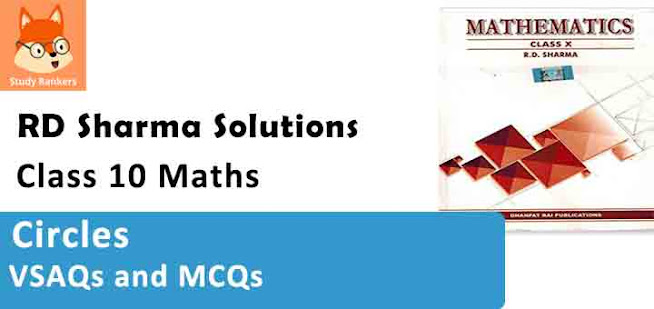#### VSAQs

Answer each of the following questions either in one word or one sentence or as per requirement of the questions :

1. In the figure, PA and PB are tangents to the circle drawn from an external point P. CD is a third tangent touching the circle at Q. If PB = 10 cm and CQ = 2 cm, what is the length PC ?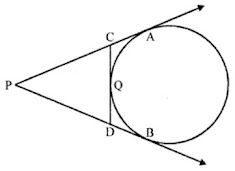Solution

In the figure, PA and PB are the tangents to the circle drawn from P
CD is the third tangent to the circle drawn at Q
PB = 10 cm, CQ = 2 cm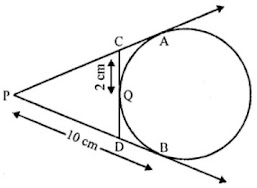PA and PB are tangents to the circle
PA = PB = 10 cm
Similarly CQ and CA are tangents to the circle
CQ = CA = 2 cm
PC = PA – CA = 10 – 2 = 8 cm

2. What is the distance between two parallel tangents of a circle of radius 4 cm ?

Solution

TT’ and SS’ are two tangents of a circle with centre O and radius 4 cm and TT’ || SS’
OP and OQ are joined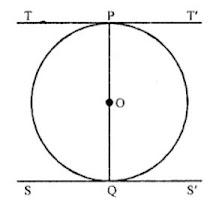Now OP is the radius and TPT’ is the tangent
OP ⊥ TPT’
Similar OQ ⊥ SS’
But TT’ || SS’
POQ is the diameter
Which is 4×2 = 8 cm
Distance between the two parallel tangents is 8 cm

3. The length of tangent from a point A at a distance of 5 cm from the centre of the circle is 4 cm. What is the radius of the circle ?

Solution

PA is a tangent to the circle from P at a distance of 5 cm from the centre O
PA = 4 cm
OA is joined and let OA = r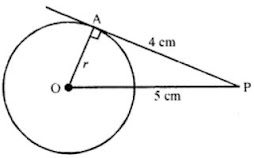Now in right ∆OAP,
OP² = OA² + PA²
⇒ (5)² = r² + (4)²
⇒ 25 = r + 16
⇒ r² = 25 – 16 = 9 = (3)²
r = 3
Radius of the circle = 3 cm

4. Two tangents TP and TQ are drawn from an external point T to a circle with centre O as shown in the following figure. If they are inclined to each other at an angle of 100°, then what is the value of ∠POQ ?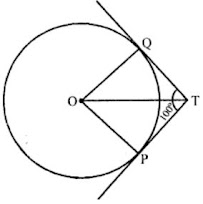Solution

TP and TQ are the tangents from T to the circle with centre O and ∠PTQ = 100°
OT, OP and OQ are joined
OP ⊥ PT and OQ ⊥ QT
∠POQ + ∠OPT + ∠PTQ + ∠OQT = 360° (Sum of angles of a quadrilateral)
⇒ ∠POQ + 90° + 100° + 90° = 360°
⇒ ∠POQ + 280° = 360°
⇒ ∠POQ = 360° – 280° = 80°
Hence ∠POQ = 80°

5. What is the distance between two parallel tangents to a circle of radius 5 cm?

Solution

In a circle, the radius is 5 cm and centre is O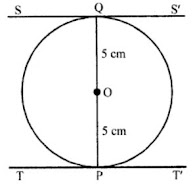TT’ and SS’ are two tangents at P and Q to the circle
Such that TT’ || SS’
Join OP and OQ
OP is radius and TPT’ is the tangent
OP ⊥ TT’
Similarly OQ ⊥ SS’
POQ is the diameter of the circle
Now length of PQ = OP + OQ = 5 + 5 = 10 cm
Hence, distance between the two parallel tangents = 10 cm

6. In Q. No. 1, if PB = 10 cm, what is the perimeter of ∆PCD ?

Solution

In the figure, PB = 10 cm, CQ = 2 cm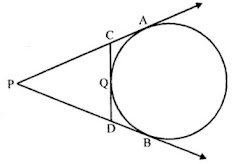PA and PB are tangents to the give from P
PA = PB = 10 cm
Similarly, CA and CQ are the tangents
CA = CQ = 2 cm
and DB and DQ are the tangents
DB = DQ
Now, perimeter of ∆PCD
PC + PD + CQ + DQ
= PC + CQ + PD + DQ
= PC + CA + PD + DB {CQ = CA and DQ = DB}
= PA + PB = 10 + 10 = 20 cm